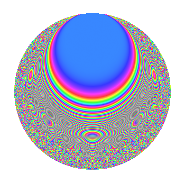# Properties

 Label 27.6.eLevel 27 Weight 6 Character orbit e Rep. character $$\chi_{27}(4,\cdot)$$ Character field $$\Q(\zeta_{9})$$ Dimension 84 Newforms 1 Sturm bound 18 Trace bound 0

# Related objects

## Defining parameters

 Level: $$N$$ = $$27 = 3^{3}$$ Weight: $$k$$ = $$6$$ Character orbit: $$[\chi]$$ = 27.e (of order $$9$$ and degree $$6$$) Character conductor: $$\operatorname{cond}(\chi)$$ = $$27$$ Character field: $$\Q(\zeta_{9})$$ Newforms: $$1$$ Sturm bound: $$18$$ Trace bound: $$0$$

## Dimensions

The following table gives the dimensions of various subspaces of $$M_{6}(27, [\chi])$$.

Total New Old
Modular forms 96 96 0
Cusp forms 84 84 0
Eisenstein series 12 12 0

## Trace form

 $$84q - 6q^{2} - 6q^{3} - 6q^{4} - 93q^{5} - 126q^{6} - 6q^{7} + 573q^{8} + 324q^{9} + O(q^{10})$$ $$84q - 6q^{2} - 6q^{3} - 6q^{4} - 93q^{5} - 126q^{6} - 6q^{7} + 573q^{8} + 324q^{9} - 3q^{10} + 111q^{11} - 2769q^{12} - 6q^{13} - 1641q^{14} + 1989q^{15} + 90q^{16} + 3465q^{17} - 99q^{18} - 3q^{19} + 9987q^{20} + 5424q^{21} - 2850q^{22} - 7716q^{23} - 18486q^{24} + 4953q^{25} - 7806q^{26} - 8109q^{27} - 12q^{28} - 20418q^{29} - 6453q^{30} - 6657q^{31} + 51192q^{32} + 50634q^{33} - 11394q^{34} + 35868q^{35} - 3600q^{36} - 3q^{37} - 44076q^{38} - 13971q^{39} + 12441q^{40} - 79077q^{41} - 64422q^{42} - 9465q^{43} + 110757q^{44} + 92493q^{45} - 3q^{46} + 103557q^{47} + 134049q^{48} + 5484q^{49} - 105513q^{50} - 124866q^{51} + 68625q^{52} - 206406q^{53} - 350946q^{54} - 12q^{55} - 147237q^{56} + 67767q^{57} - 9753q^{58} + 116484q^{59} + 241290q^{60} - 70116q^{61} + 246066q^{62} + 135981q^{63} - 86019q^{64} - 19815q^{65} - 32931q^{66} + 48117q^{67} - 48105q^{68} + 25263q^{69} + 270111q^{70} + 279531q^{71} + 441684q^{72} - 27012q^{73} + 233691q^{74} + 18399q^{75} - 125670q^{76} - 345135q^{77} - 520974q^{78} - 216186q^{79} - 924114q^{80} - 428616q^{81} - 12q^{82} - 370401q^{83} - 522390q^{84} - 43731q^{85} + 116682q^{86} + 304173q^{87} + 371418q^{88} + 154827q^{89} + 327366q^{90} - 91002q^{91} + 1279059q^{92} + 890079q^{93} + 11667q^{94} + 1087671q^{95} + 1165698q^{96} - 420621q^{97} + 463410q^{98} - 28323q^{99} + O(q^{100})$$

## Decomposition of $$S_{6}^{\mathrm{new}}(27, [\chi])$$ into irreducible Hecke orbits

Label Dim. $$A$$ Field CM Traces $q$-expansion
$$a_2$$ $$a_3$$ $$a_5$$ $$a_7$$
27.6.e.a $$84$$ $$4.330$$ None $$-6$$ $$-6$$ $$-93$$ $$-6$$